Numbers’ Magic

# Numbers’ Magic - Notes | Study General Knowledge Olympiad for Class 1 - Class 1

 1 Crore+ students have signed up on EduRev. Have you?

Maths is important to develop vital life skills and our own spatial awareness. In this way, this chapter gets students acquainted with counting numbers from 1 to 20 and learn basic mathematical problems like subtraction and addition. This also focuses on recognising various shapes and sizes of objects, actual time, currency, etc. This chapter makes you aware of the physical attributes of objects and develops skills recognising patterns in your environment.

Number: It is a mathematical unit used to count, label or measure.

Number words: Number words are representation of numbers in language.

Representation of numbers from 1 to 20 in words

Concept of Ones, Tens, and Hundreds

These are places of digits in a number.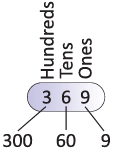It is the process of calculating total or sum of two or more values, digits or numbers.
To find the total number of one-digit numbers:

• Count the first number on your fingers.
• Count the second number on your fingers.
• The total number of fingers is the result.

For Example: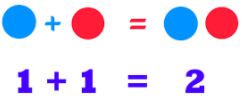Subtraction

It is the process of calculating how many are left or how many more or less.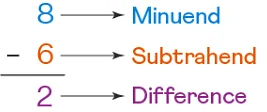Geometrical Shapes

A geometric shape is a figure closed by combining points, lines or curves. There are four basic geometric shapes which are: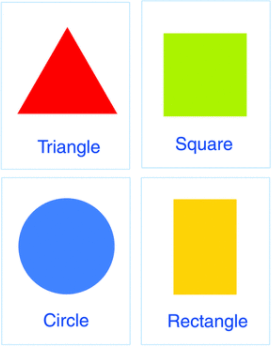• Circle: A perfect round shape.
• Square: A figure with four equal sides.
• Rectangle: A figure with four sides, in which opposite sides are parallel and equal.
• Triangle: A figure with three sides linked end-to-end.

Money

Money is a medium of exchange, i.e., buying and selling of objects.

• Coins currently used in India: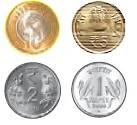• Paper Notes used in India: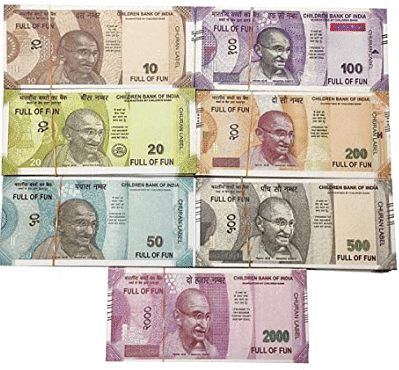Weight

Weight is a quantity or amount of mass in an object.

• Heavy Objects: Things which have more weight are called heavy. Usually heavy things are large in size and hard.  For example, truck, car, scooter, bed, almirah, etc.
• Light Objects: Things which have less weight are called light. Light things are easy to carry. For example, feather, leaf, paper, pencil, etc.
• Comparing Heavy and Light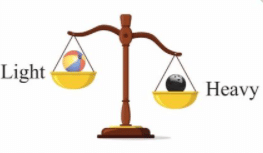Length

Length is a measurement which tells how long an object is.

• Long or Tall Object: An object which has more height or length is called a tall or long object. For example, train, tree, bamboo, etc.
• Short Object: An object which has less height or length is called a short object. For example, eraser, bowl, etc.
• Comparing Tall and Short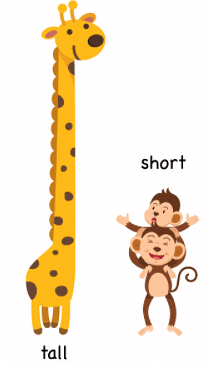Time

• Measuring units of time are seconds, minutes and hours. For example, 3 hours; 20 minutes; 40 seconds.
• When we measure time, we measure the duration or period of a certain event. For example, Meera studied for 3 hours 45 minutes.
• Now just remember:
• 24 hours = 1 day
• 1 hour = 60 minutes
• 1 minute = 60 seconds
• Clock Indicating Time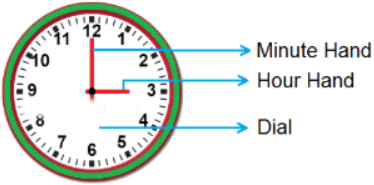• When a minute hand is at 12, then the actual time is the number that is indicated by the hour hand. For example, the given clock indicates that the time is 9 O’ Clock as minute hand is at 12 and hour hand is at 9.
• If hour hand is somewhere between 2 numbers, for example, between 9 and 10, minute hand is at a particular number, for example, at , and the time is 9:2 and it will be read as 2 minutes past 9.

Calendar

• It is a systematic arrangement of days, weeks, months and year to show various periods of time.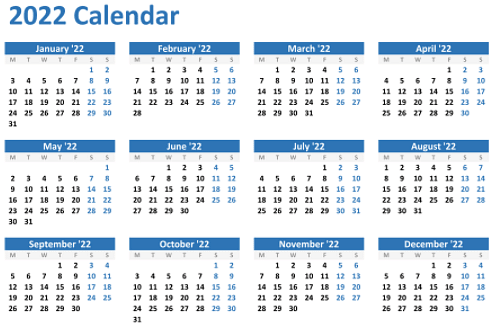• Days in a Week: A week consists of seven days which are as follows:
• Monday
• Tuesday
• Wednesday
• Thursday
• Friday
• Saturday
• Sunday
• Months in a Year: There are 12 months in a year, which are:
• January
• February
• March
• April
• May
• June
• July
• August
• September
• October
• November
• December
• Patterns: This is all about- numbers, shapes, images- that repeat in a logical manner. One needs to make logical connections in the given pattern to understand what comes next.
The document Numbers’ Magic - Notes | Study General Knowledge Olympiad for Class 1 - Class 1 is a part of the Class 1 Course General Knowledge Olympiad for Class 1.
All you need of Class 1 at this link: Class 1

## General Knowledge Olympiad for Class 1

20 docs|39 tests
 Use Code STAYHOME200 and get INR 200 additional OFF

## General Knowledge Olympiad for Class 1

20 docs|39 tests

Track your progress, build streaks, highlight & save important lessons and more!

,

,

,

,

,

,

,

,

,

,

,

,

,

,

,

,

,

,

,

,

,

;